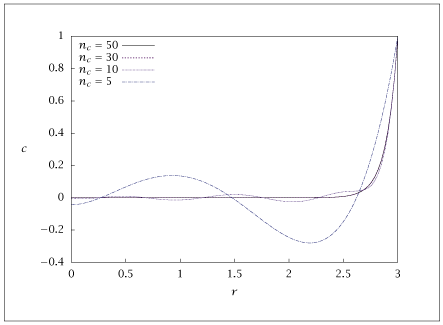## Figure A.5:

### Dimensionless concentration versus dimensionless radial position for different numbers of collocation points.## Code for Figure A.5

### main.m

```
1
2
3
4
5
6
7
8
9
10
11
12
13
14
15
16
17
18
19
20
21
22
23
24
25
26
27
28
29
30
31
32
33
34
35
36
37
38
39
40
41
42
43
44
45
46
47
48
49
50
51
52
53
54
55
56
57
58
59
60
61
62
63
64
65
66
67
68
69
70
71
72
73
74% Copyright (C) 2001, James B. Rawlings and John G. Ekerdt
%
% This program is free software; you can redistribute it and/or
% modify it under the terms of the GNU General Public License as
% published by the Free Software Foundation; either version 2, or (at
% your option) any later version.
%
% This program is distributed in the hope that it will be useful, but
% WITHOUT ANY WARRANTY; without even the implied warranty of
% MERCHANTABILITY or FITNESS FOR A PARTICULAR PURPOSE.  See the GNU
% General Public License for more details.
%
% You should have received a copy of the GNU General Public License
% along with this program; see the file COPYING.  If not, write to
% the Free Software Foundation, 59 Temple Place - Suite 330, Boston,
% MA 02111-1307, USA.

Phi  = 10;
n    = 1;
p.Phi = Phi;
p.n = n;

nint = 100;
xint = linspace(0, 3, nint)';

colvec = [5; 10; 30; 50];
ncol   = length(colvec);
ccol   = zeros(ncol, ncol);
csave  = zeros(nint, ncol);
cerr   = zeros(nint, ncol);
can    = 3./xint.*sinh(Phi*xint)/sinh(3*Phi);
can(1) = 3*Phi/sinh(3*Phi);
etaan = 1./Phi*(1./tanh(3*Phi)-1/(3*Phi));
for i = 1: ncol
npts = colvec(i);
%
% collocation
%
[R A B Q] = colloc(npts-2, 'left', 'right');
R = R*3;
A = A/3;
B = B/9;
Q = Q*3;
p.R = R;
p.A = A;
p.B = B;
W = polinterp(R,xint);
%
% solve the problem
%
c0=0.5*linspace(0,1,npts)';
tol = 1e-10;
opts = optimset ('TolFun', tol);
[c,fval,info] = fsolve(@(x) pellet(x,p), c0, opts);
cint = W*c;
csave(:,i) = cint;
cerr(:,i)  = abs((cint - can))./can;
%
% compute the effectiveness factor
%
eta  = (n+1)/2*A(npts,:)*c/Phi^2;
etaerr(i) = abs(eta - etaan)/etaan;
results = [R c];
%  save ('-ascii', sprintf ('pelletcolloc_%d.dat', i), 'results');
end
table = [xint csave cerr];
save -ascii pelletcolloc.dat table;

if (~ strcmp (getenv ('OMIT_PLOTS'), 'true')) % PLOTTING
%plot the pellet profiles for different collocation numbers
plot(xint, csave(:,1:ncol))
% TITLE
end % PLOTTING

```

### polinterp.m

```
1
2
3
4
5
6
7
8
9
10
11
12
13
14
15
16
17
18
19
20
21
22function W = polinterp(xcol,xint)
ncol = length(xcol);
nint = length(xint);
W = zeros(nint, ncol);
for i = 1: ncol
d = 1.0;
for j = 1: ncol
if (j ~= i )
d = d*(xcol(i) - xcol(j));
end
end
for k = 1: nint
xx = xint(k);
n = 1.0;
for j = 1: ncol
if (j ~= i)
n = n*(xx- xcol(j));
end
end
W(k,i) = n/d;
end
end

```

### pellet.m

```
1
2
3
4function retval = pellet(c, p)
retval = p.B*c + 2*p.A*c./p.R - 2/(p.n+1)*p.Phi^2*(c.^p.n);
retval(1)    = p.A(1,:)*c;
retval(end) = 1 - c(end);

```